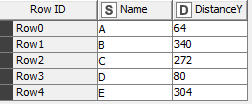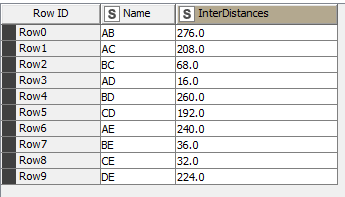# Labeling combinations from Distance Matrix Calculate node

I didn’t find a specific answer to this problem on the forums and I hope someone here can help me out.

I’m using the Distance Matrix Calculate node to get all distances between a series of values. My problem is that I want to get a label for each distance that is a string composed of a combination of the name of the two individual values. For example for these five values:I want this end result:Calculating the distance matrix is easy but I haven’t been able to figure out how to use the split value information to generate specific IDs. I know that this is possible to do manually with loops but the distance matrix calculate node is much more efficient when dealing with large numbers as well as duplicate combinations not being an issue.

Here is a link to workflow with this problem and I would be very grateful if anyone knows how to solve it.

If you put a Distance Matrix Pair Extractor node after the Distance Matrix Calculate node, you’ll get the information you need:From here you can create your label using a String Manipulation node to join the contents of the Object1 and Object2 columns.

2 Likes

Thanks so much @elsamuel! That’s super useful.

This topic was automatically closed 7 days after the last reply. New replies are no longer allowed.